Solving homogeneous differential equation

Chapter 9 Class 12 Differential Equations
Concept wise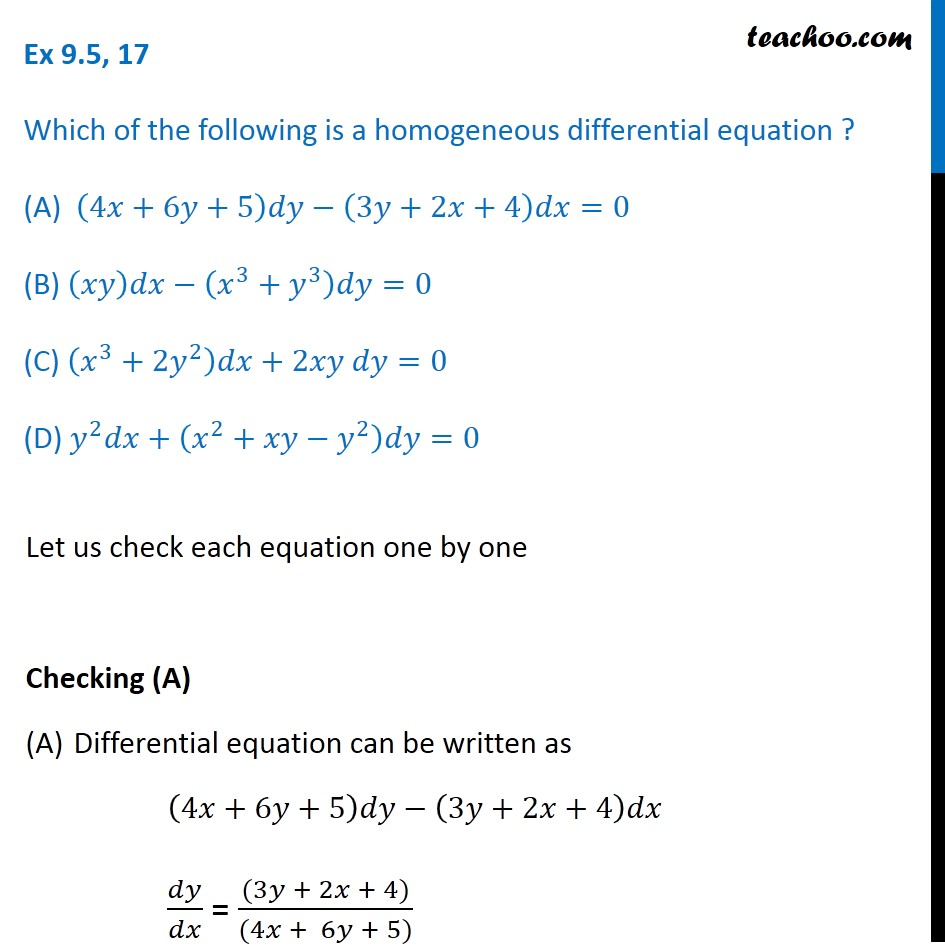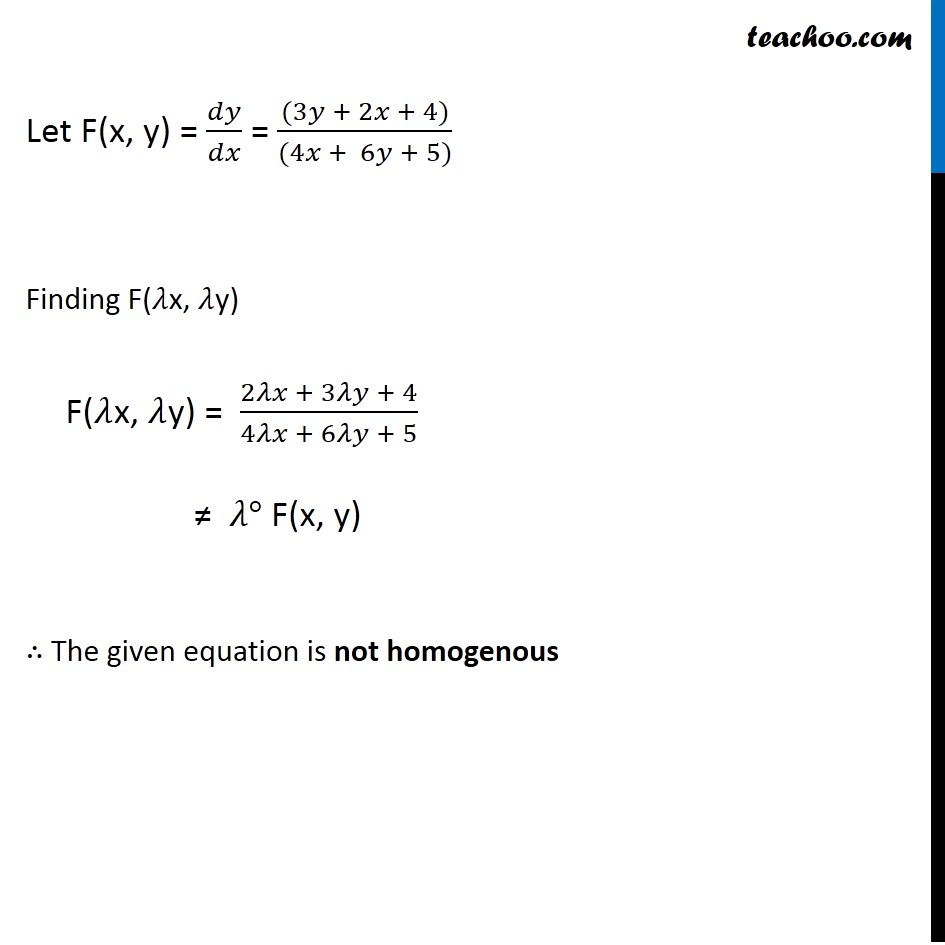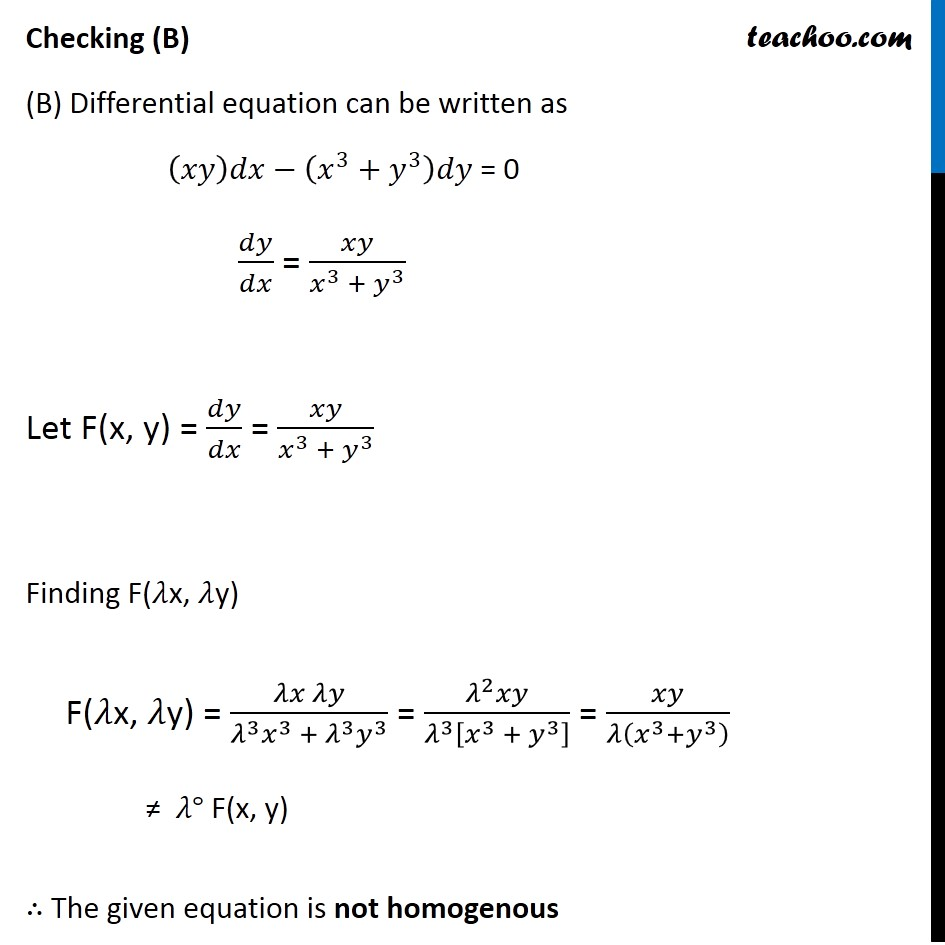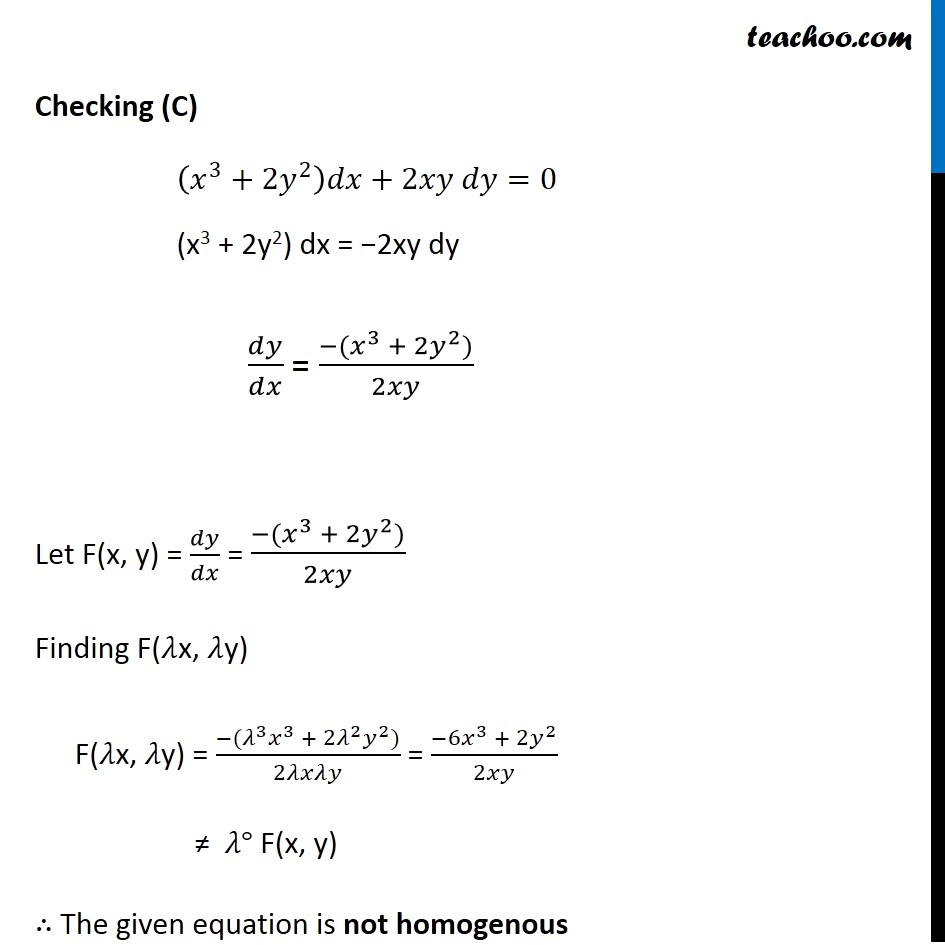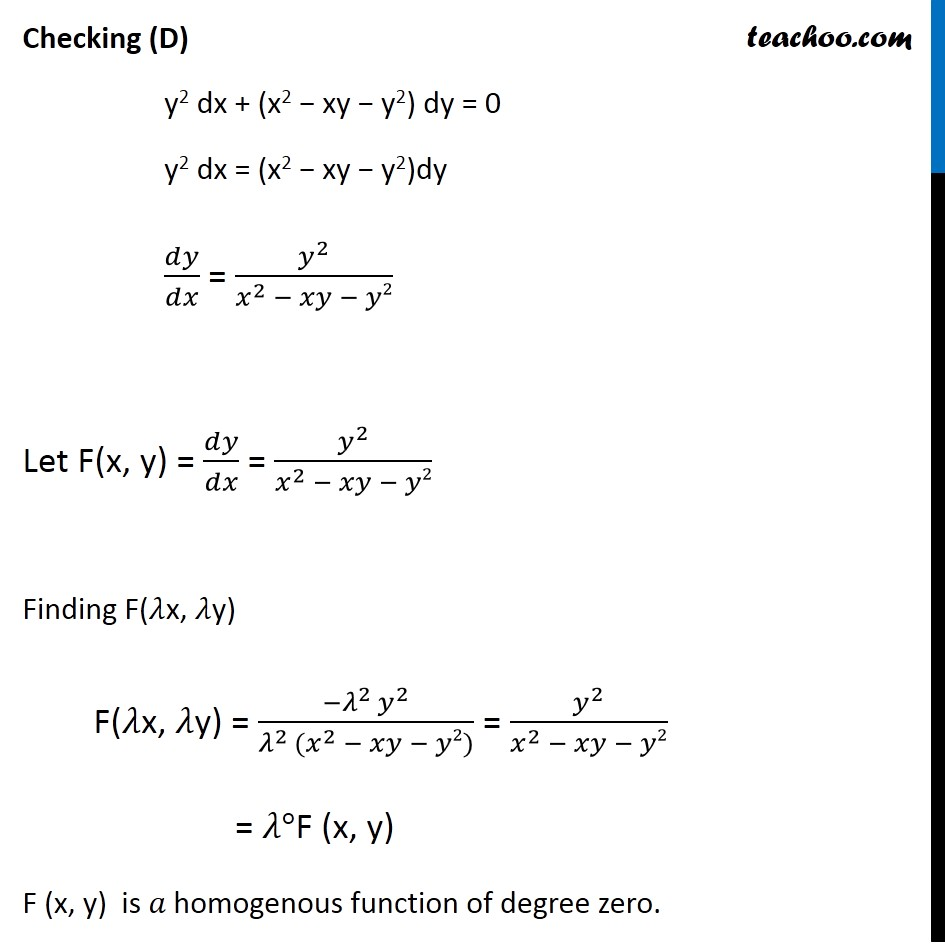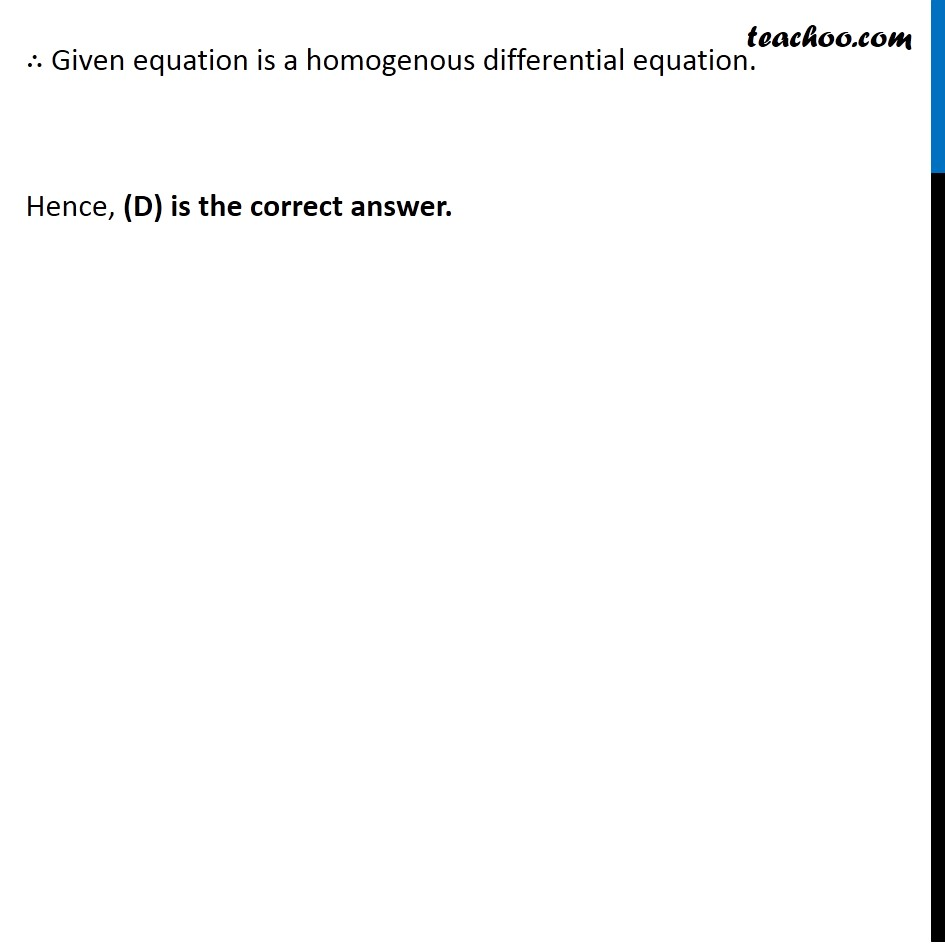Introducing your new favourite teacher - Teachoo Black, at only ₹83 per month

### Transcript

Ex 9.5, 17 Which of the following is a homogeneous differential equation ? (A) (4𝑥+6𝑦+5)𝑑𝑦−(3𝑦+2𝑥+4)𝑑𝑥=0 (B) (𝑥𝑦)𝑑𝑥−(𝑥^3+𝑦^3 )𝑑𝑦=0 (C) (𝑥^3+2𝑦^2 )𝑑𝑥+2𝑥𝑦 𝑑𝑦=0 (D) 𝑦^2 𝑑𝑥+(𝑥^2+𝑥𝑦−𝑦^2 )𝑑𝑦=0 Let us check each equation one by one Checking (A) Differential equation can be written as (4𝑥+6𝑦+5)𝑑𝑦−(3𝑦+2𝑥+4)𝑑𝑥 𝑑𝑦/𝑑𝑥 = ((3𝑦 + 2𝑥 + 4))/((4𝑥 + 6𝑦 + 5)) Let F(x, y) = 𝑑𝑦/𝑑𝑥 = ((3𝑦 + 2𝑥 + 4))/((4𝑥 + 6𝑦 + 5)) Finding F(𝜆x, 𝜆y) F(𝜆x, 𝜆y) = (2𝜆𝑥 + 3𝜆𝑦 + 4)/(4𝜆𝑥 + 6𝜆𝑦 + 5) ≠ 𝜆° F(x, y) ∴ The given equation is not homogenous Checking (B) (B) Differential equation can be written as (𝑥𝑦)𝑑𝑥−(𝑥^3+𝑦^3 )𝑑𝑦 = 0 𝑑𝑦/𝑑𝑥 = 𝑥𝑦/(𝑥^3 + 𝑦^3 ) Let F(x, y) = 𝑑𝑦/𝑑𝑥 = 𝑥𝑦/(𝑥^3 + 𝑦^3 ) Finding F(𝜆x, 𝜆y) F(𝜆x, 𝜆y) = (𝜆𝑥 𝜆𝑦)/(𝜆^3 𝑥^3 + 𝜆^3 𝑦^3 ) = (𝜆^2 𝑥𝑦)/(𝜆^3 [𝑥^3 + 𝑦^3 ] ) = 𝑥𝑦/𝜆(𝑥^3+𝑦^3 ) ≠ 𝜆° F(x, y) ∴ The given equation is not homogenous Checking (C) (𝑥^3+2𝑦^2 )𝑑𝑥+2𝑥𝑦 𝑑𝑦=0 (x3 + 2y2) dx = −2xy dy 𝑑𝑦/𝑑𝑥 = (−(𝑥^3 + 2𝑦^2))/2𝑥𝑦 Let F(x, y) = 𝑑𝑦/𝑑𝑥 = (−(𝑥^3 + 2𝑦^2))/2𝑥𝑦 Finding F(𝜆x, 𝜆y) F(𝜆x, 𝜆y) = (−(𝜆^3 𝑥^3 + 2𝜆^2 𝑦^2))/2𝜆𝑥𝜆𝑦 = (−〖6𝑥〗^3 + 2𝑦^2)/2𝑥𝑦 ≠ 𝜆° F(x, y) ∴ The given equation is not homogenous Checking (D) y2 dx + (x2 − xy − y2) dy = 0 y2 dx = (x2 − xy − y2)dy 𝑑𝑦/𝑑𝑥 = 𝑦^2/(𝑥^2 − 𝑥𝑦 − 𝑦2) Let F(x, y) = 𝑑𝑦/𝑑𝑥 = 𝑦^2/(𝑥^2 − 𝑥𝑦 − 𝑦2) Finding F(𝜆x, 𝜆y) F(𝜆x, 𝜆y) = 〖−𝜆^(2 ) 𝑦〗^2/(𝜆^(2 ) (𝑥^2 − 𝑥𝑦 − 𝑦2)) = 𝑦^2/(𝑥^2 − 𝑥𝑦 − 𝑦2) = 𝜆°F (x, y) F (x, y) is 𝑎 homogenous function of degree zero. ∴ Given equation is a homogenous differential equation. Hence, (D) is the correct answer.